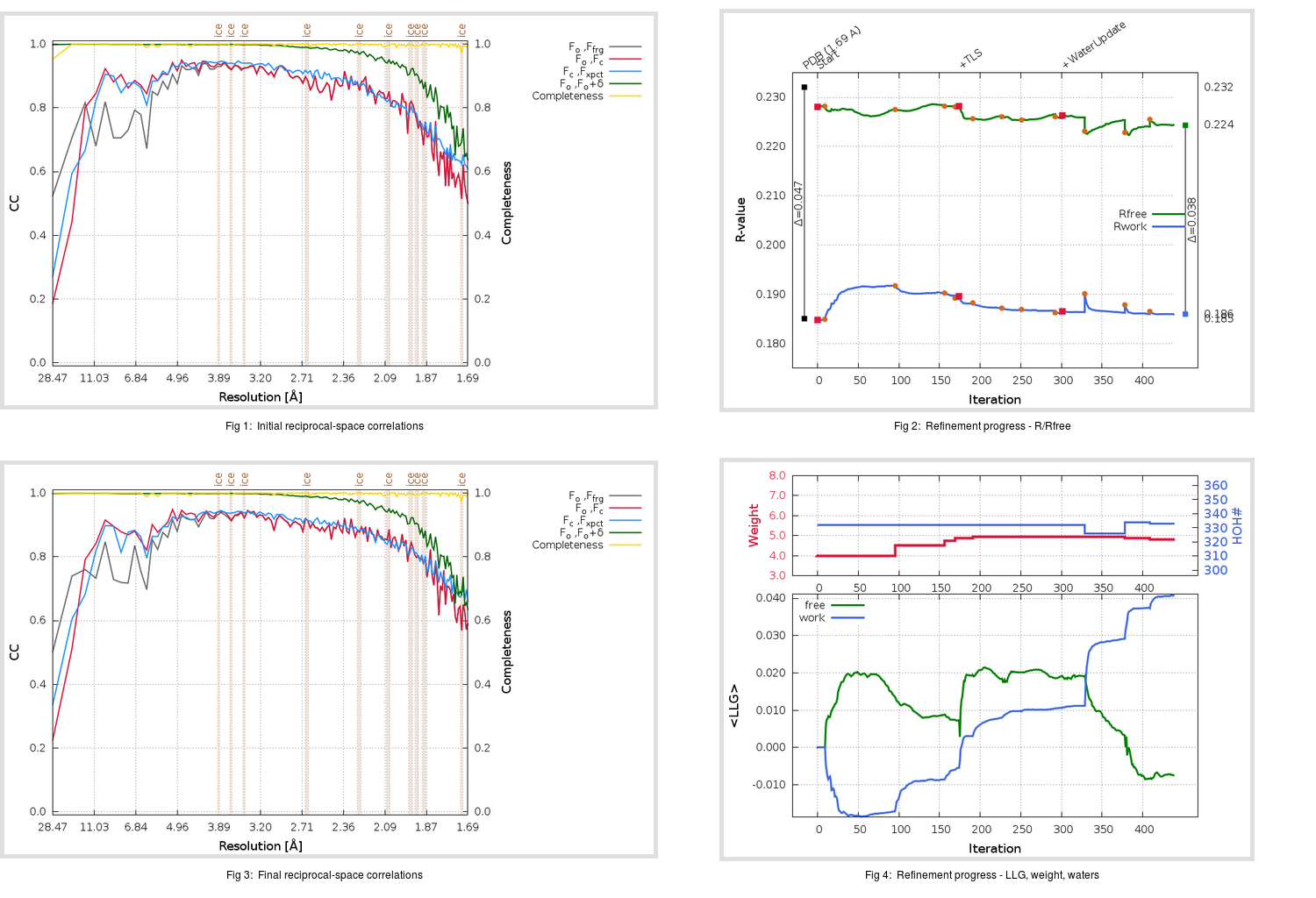Content:

```    Diffraction limits & principal axes of ellipsoid fitted to diffraction cut-off surface:
1.712         0.9950   0.0000   0.0999       0.999 a* - 0.050 c*
1.651         0.0000   1.0000   0.0000       b*
1.628        -0.0999   0.0000   0.9950      -0.246 a* + 0.969 c*
```

## Deposited

` `
 Date deposited Date data collection Resolution R, Rfree 20200315 20200304 1.69 0.1830 0.2320

Molprobity (CCP4 7.0 version) summary:

```Ramachandran outliers =   0.33 %
favored =  97.68 %
Rotamer outliers      =   0.76 %
C-beta deviations     =     0
Clashscore            =   5.05
RMS(bonds)            =   0.0131
RMS(angles)           =   1.84
MolProbity score      =   1.34
Resolution            =   1.69
R-work                =   0.1830
R-free                =   0.2320
```

```Number of waters      =   332

<B> (all atoms) =   23.54 ( sd =    9.35 ) for       2738 non-hydrogen atoms
<B>   (protein) =   22.13 ( sd =    8.58 ) for       2370 non-hydrogen atoms
<B>     (water) =   32.97 ( sd =    9.17 ) for        332 non-hydrogen atoms
<B>    (others) =   29.61 ( sd =   10.24 ) for         36 non-hydrogen atoms

B min/max       (all non-hydrogen atoms) =   12.24 /   74.87
B min/max   (protein non-hydrogen atoms) =   12.24 /   74.87
B min/max     (water non-hydrogen atoms) =   12.91 /   64.74
B min/max     (other non-hydrogen atoms) =   20.65 /   41.64
```

## BUSTER (re-)refinement

` `

Molprobity (CCP4 7.0 version) summary:

```Ramachandran outliers =   0.33 %
favored =  98.34 %
Rotamer outliers      =   1.89 %
C-beta deviations     =     0
Clashscore            =   1.90
RMS(bonds)            =   0.0116
RMS(angles)           =   1.59
MolProbity score      =   1.16
Resolution            =   1.69
R-work                =   0.1860
R-free                =   0.2244
```

```Number of waters      =   333

<B> (all atoms) =   24.18 ( sd =    8.96 ) for       2739 non-hydrogen atoms
<B>   (protein) =   22.44 ( sd =    7.24 ) for       2370 non-hydrogen atoms
<B>     (water) =   35.95 ( sd =   10.77 ) for        333 non-hydrogen atoms
<B>    (others) =   30.04 ( sd =   10.69 ) for         36 non-hydrogen atoms

B min/max       (all non-hydrogen atoms) =   11.08 /   75.28
B min/max   (protein non-hydrogen atoms) =   11.08 /   60.59
B min/max     (water non-hydrogen atoms) =   13.25 /   75.28
B min/max     (other non-hydrogen atoms) =   23.19 /   41.30
```

Refinement progression:Results:

` `
 File Remark 5REU_aB_refine.01_03_refine.pdb.gz exact refinement commands are in header 5REU_aB_refine.01_03_refine.mtz.gz including original deposited data and several re-refinement map coefficients 5REU_aB_refine.01_03_BUSTER_model.cif.gz including any non-standard compound restraints 5REU_aB_refine.01_03_BUSTER_refln.cif.gz